### DJ dogHover over the thumbnail for a full-size version.

Author ultimatereading author:ultimatereading n-art nart rated 2010-05-21 2010-05-21 4 by 13 people. \$DJ dog#ultimatereading#nart#00000000000000000000000000000000000000000000000000000000000000000000000000000000000000000000000000000000000000000000000000000000000000000000000000000000000000000000000000000000000000000000000000000000000000000000000000000000000000000000000000000000000000000000000000000000000000000000000000000000000000000000000000000000000000000000000000000000000000000000000000000000000000000000000000000000000000000000000000000000000000000000000000000000000000000000000000000000000000000000000000000000000000000000000000000000000000000000000000000000000000000000000000000000000000000000000000000000000000000000000000000000000000000000000000000000000000000000000000000000000000000000000000000000000000000000000000000000000000000|12^318,146!12^319,138!12^318,142!12^320,134!12^320,130!12^321,128!12^322,123!12^325,126!12^329,132!12^328,130!12^332,136!12^335,143!12^333,139!12^314,149!12^308,151!12^309,151!12^305,150!12^300,147!12^298,143!12^296,141!12^292,138!12^303,148!12^289,136!12^287,134!12^284,129!12^283,129!12^282,131!12^283,137!12^284,145!12^284,142!12^285,150!12^285,155!12^287,161!12^286,159!12^289,164!12^292,171!12^291,170!12^290,170!12^294,176!12^293,175!12^297,181!12^298,186!12^300,188!12^303,194!12^300,188!12^304,194!12^306,199!12^306,202!12^306,207!12^305,212!12^304,217!12^300,221!12^296,224!12^292,227!12^288,230!12^284,234!12^279,237!12^274,239!12^269,243!12^271,241!12^266,245!12^262,248!12^258,251!12^254,255!12^249,256!12^245,255!12^242,251!12^240,250!12^236,248!12^235,246!12^230,241!12^232,244!12^338,145!12^342,143!12^347,140!12^354,139!12^345,142!12^350,139!12^353,137!12^358,135!12^363,133!12^362,135!12^226,238!12^220,233!12^225,238!12^223,236!12^367,131!12^371,129!12^376,126!12^375,127!12^289,133!12^217,229!12^214,224!12^210,221!12^208,218!12^206,216!12^205,214!12^203,211!12^199,208!12^199,204!12^199,198!12^200,192!12^199,187!12^196,184!12^192,183!12^187,184!12^182,187!12^178,192!12^176,198!12^176,206!12^176,203!12^177,194!12^181,188!12^176,212!12^179,218!12^185,226!12^182,225!12^181,223!12^178,220!12^177,216!12^187,230!12^190,234!12^195,239!12^196,241!12^193,239!12^199,246!12^203,251!12^206,254!12^211,259!12^216,265!12^212,261!12^205,254!12^222,271!12^219,269!12^210,258!12^227,275!12^232,279!12^237,284!12^234,281!12^229,276!12^225,272!12^239,286!12^242,288!12^246,291!12^248,293!12^254,291!12^250,294!12^256,288!12^259,286!12^262,285!12^265,283!12^269,280!12^275,277!12^272,278!12^278,274!12^284,271!12^287,267!12^380,124!12^384,122!12^388,120!12^282,271!12^292,265!12^298,262!12^295,263!12^301,258!12^307,255!12^305,257!12^307,260!12^307,267!12^308,267!12^307,272!12^308,278!12^307,284!12^308,289!12^308,295!12^308,300!12^307,305!0^204,180!0^210,156!0^180,126!0^150,162!0^162,186!0^174,127!0^169,128!0^165,130!0^154,136!0^152,141!0^156,176!0^201,136!0^186,127!0^200,135!0^197,132!0^193,129!0^164,130!0^160,133!0^151,145!0^150,151!0^150,157!0^152,170!0^151,168!0^156,176!0^156,174!0^159,180!0^163,185!0^169,188!0^211,154!0^210,148!0^208,144!0^205,139!0^212,162!0^211,167!0^209,172!0^207,179!0^203,182!0^174,191!0^180,150!0^175,153!0^186,153!0^189,161!0^189,159!0^177,170!0^174,167!0^172,163!0^173,158!0^185,171!0^186,167!0^183,170!12^392,119!12^396,117!12^399,116!12^404,121!12^404,119!12^407,126!12^410,130!12^411,136!12^405,139!12^406,140!12^402,142!12^395,146!12^397,145!12^390,150!12^386,151!12^382,154!12^391,148!12^376,157!12^380,155!12^369,160!12^373,159!12^372,163!12^379,164!12^383,164!12^398,166!12^393,167!12^404,166!12^402,166!12^411,167!12^409,167!12^416,168!12^418,176!12^418,182!12^418,180!12^386,166!12^390,166!12^416,189!12^417,189!12^416,188!12^417,174!12^412,189!12^406,189!12^401,188!12^395,188!12^389,187!12^383,186!12^377,186!12^373,186!12^369,187!12^369,193!12^368,200!12^369,193!12^369,199!12^368,205!12^373,207!12^379,209!12^385,212!12^383,211!12^390,215!12^396,217!12^402,220!12^407,222!12^398,219!12^415,226!12^411,224!12^420,228!12^426,231!12^424,230!12^308,311!12^307,316!0^306,324!0^318,324!0^330,324!0^342,330!0^312,325!0^321,325!0^326,323!0^335,326!0^341,328!0^300,324!0^294,324!0^288,324!0^282,324!0^270,330!0^284,326!0^289,326!0^295,326!0^298,326!0^303,326!0^306,327!0^310,327!0^313,326!0^278,326!0^274,328!0^345,332!0^350,338!0^347,344!0^341,348!0^335,350!0^348,335!0^349,343!0^345,348!0^337,352!0^267,331!0^262,335!0^264,341!0^332,351!0^325,353!0^319,353!0^313,353!0^311,353!0^306,353!0^332,353!0^267,346!0^273,349!0^281,351!0^291,352!0^298,352!0^293,352!0^289,352!0^286,352!0^282,352!0^279,350!0^302,353!0^272,348!0^296,338!0^301,340!0^305,339!0^311,340!0^316,340!3^259,347!3^253,346!3^245,347!3^242,348!3^240,348!3^250,349!3^232,348!3^225,347!3^225,355!3^225,365!3^225,359!3^224,353!3^225,347!3^224,369!3^225,373!3^224,375!3^233,377!3^236,376!3^243,377!3^237,376!3^232,377!3^228,376!3^249,378!3^255,378!3^261,378!3^268,377!3^276,378!3^358,342!3^364,343!3^360,342!3^368,344!3^361,376!3^353,376!3^348,377!3^347,377!3^343,377!3^336,377!3^334,378!3^331,378!3^328,377!3^325,377!3^320,378!3^313,379!3^310,378!3^282,377!3^288,377!3^293,378!3^299,378!3^305,377!3^254,345!3^252,346!3^236,347!3^232,347!3^230,348!3^229,348!3^226,351!3^241,376!3^250,377!3^259,378!3^266,378!3^274,378!3^278,379!3^289,380!3^295,379!3^302,378!3^309,377!3^361,376!3^357,376!3^357,376!3^352,376!10^211,367!10^205,366!10^200,368!10^200,368!10^195,367!10^192,367!10^191,368!10^184,368!10^184,368!10^176,368!10^167,369!10^163,369!10^162,369!10^173,367!10^214,366!10^214,368!10^157,368!10^156,374!10^156,384!10^156,384!10^156,396!10^156,390!10^156,378!10^156,402!10^156,396!10^156,408!10^156,408!10^156,402!10^156,414!10^156,426!10^156,426!10^156,420!10^154,377!10^154,384!10^154,388!10^155,396!10^155,403!10^157,416!10^157,427!10^180,426!10^180,414!10^180,420!10^180,420!10^180,432!10^180,426!10^180,408!10^180,402!10^180,396!10^192,396!10^186,396!12^431,232!10^155,416!10^156,426!10^181,432!10^181,424!10^181,419!10^180,414!10^180,410!10^180,397!10^187,397!10^192,397!10^198,395!10^204,395!10^196,396!10^208,397!10^215,396!10^210,395!10^213,395!10^219,395!10^219,395!10^221,396!10^223,396!10^228,396!10^234,396!10^240,396!10^252,396!10^246,396!10^258,396!10^264,396!10^270,396!10^276,396!10^282,396!10^288,396!10^300,396!10^294,396!10^306,396!10^312,396!10^318,396!10^324,396!10^237,395!10^246,395!10^252,396!10^256,396!10^263,396!10^271,396!10^274,397!10^278,397!10^283,397!10^288,397!10^295,397!10^306,398!10^317,399!10^324,399!10^229,398!10^241,399!10^247,400!10^156,372!10^157,386!10^157,402!10^157,421!10^157,430!10^159,367!10^166,366!10^171,368!10^178,366!10^185,366!10^195,366!10^200,366!10^179,397!10^179,410!10^179,416!10^180,426!10^258,396!10^273,396!10^290,397!10^330,396!10^342,396!10^336,396!10^348,396!10^348,396!10^360,396!10^354,396!10^366,396!10^384,396!10^378,396!10^372,396!10^390,396!10^396,396!10^402,396!10^408,396!10^420,396!10^414,396!10^329,395!10^335,396!10^339,396!10^344,394!10^348,396!10^355,396!10^362,396!10^367,395!10^370,397!10^374,397!10^384,396!10^393,396!10^401,397!10^413,396!10^420,397!3^402,342!3^402,348!3^402,354!3^402,360!3^402,366!3^402,336!3^408,336!3^414,336!3^414,336!3^408,336!3^420,336!3^421,336!3^417,337!3^415,337!3^413,337!3^412,337!3^409,337!3^409,337!3^409,337!3^406,338!3^406,342!3^405,349!3^404,354!3^405,361!3^405,361!3^405,366!0^432,336!0^435,333!0^440,328!0^446,325!0^433,341!0^474,324!0^480,324!0^486,324!0^492,324!0^498,324!0^516,336!0^462,354!0^437,345!0^443,349!0^448,352!0^455,353!0^478,323!0^481,323!0^487,325!0^492,325!0^496,327!0^503,326!0^516,335!0^513,330!0^509,327!0^505,325!0^517,340!0^514,346!0^509,351!0^501,354!0^470,356!0^477,356!0^485,356!0^491,356!0^494,357!0^468,355!0^470,339!0^487,339!0^476,340!0^480,343!0^481,340!0^483,339!3^414,372!3^420,372!3^432,372!3^403,372!3^405,374!3^411,374!3^415,374!3^416,374!3^419,374!3^423,375!3^403,375!3^432,374!3^439,372!3^445,374!3^449,374!3^436,375!3^427,375!3^443,373!3^446,374!3^453,374!3^456,372!12^437,237!12^435,236!12^442,239!12^446,246!12^445,243!12^447,250!12^449,257!12^450,262!12^451,267!12^452,269!12^453,277!12^452,274!12^447,254!12^454,283!12^456,289!12^453,285!12^455,293!12^457,299!12^456,297!12^459,305!12^462,309!12^468,313!12^467,312!12^471,316!12^475,322!12^476,330!12^472,334!12^476,325!12^465,336!12^462,333!12^469,337!12^459,331!12^453,326!12^451,323!12^450,317!12^447,314!12^445,310!12^443,306!12^440,302!12^438,295!12^436,288!12^439,300!12^435,283!12^437,292!12^434,279!12^433,273!12^430,267!12^430,263!12^432,269!12^429,259!12^424,257!12^420,256!12^416,252!12^422,255!12^412,250!12^408,248!12^404,246!12^400,244!12^397,243!12^394,242!12^391,240!12^387,237!12^383,237!12^380,234!12^374,233!12^373,239!12^373,243!12^374,249!12^374,254!12^375,259!12^374,267!12^375,263!12^376,272!12^376,277!12^376,282!12^377,286!12^376,268!12^377,291!12^378,296!12^378,303!12^378,299!12^378,311!12^378,306!12^378,318!12^378,315!12^378,324!12^378,322!12^378,331!12^378,329!12^378,337!12^378,336!10^167,430!10^172,431!10^172,430!10^170,430!10^166,431!10^164,431!10^182,432!3^377,342!3^373,340!3^365,377!3^372,377!3^377,345!3^377,352!3^378,359!3^378,366!3^378,373!3^377,377!3^357,341!3^363,340!3^364,340!3^368,342!3^370,342!3^371,342!3^371,342!3^375,343!3^378,342!3^378,348!3^378,349!3^378,352!3^379,355!3^379,356!3^379,359!3^380,361!3^380,362!3^380,366!3^380,367!3^380,371!3^380,372!3^381,374!3^373,377!3^368,377!3^363,378!3^357,378!3^352,378!3^456,375!3^461,375!3^465,373!3^468,372!3^474,372!3^480,372!3^486,372!3^492,372!3^498,372!3^510,372!3^516,372!3^504,372!3^522,372!3^528,372!3^522,372!10^426,396!10^432,396!10^438,396!10^444,396!10^450,396!10^462,396!10^456,396!10^468,396!10^474,396!10^480,396!10^480,396!10^486,396!10^492,396!10^504,396!10^498,396!10^510,396!10^516,396!10^516,396!10^522,396!10^528,396!10^534,396!10^540,396!10^546,396!10^546,402!10^546,408!10^546,414!10^546,420!10^546,426!10^546,432!10^558,432!10^570,432!10^570,420!10^570,408!10^570,396!10^570,384!10^552,432!10^564,432!10^570,426!10^570,414!10^570,402!10^570,390!10^417,396!10^424,396!10^430,396!10^436,395!10^441,394!10^447,393!10^453,395!10^457,396!10^462,396!10^468,398!10^476,397!10^480,397!10^488,396!10^495,396!10^495,394!10^502,394!10^506,394!10^548,397!10^541,396!10^537,394!10^528,394!10^521,393!10^509,394!10^547,403!10^547,410!10^546,415!10^546,422!10^547,427!10^548,434!10^555,434!10^561,432!10^568,433!10^569,434!10^573,423!10^573,415!10^571,404!10^571,396!10^571,389!10^571,386!3^466,373!3^472,373!3^477,374!3^480,375!3^482,373!3^487,374!3^488,374!3^498,375!3^504,377!3^508,377!3^515,374!3^520,374!3^525,374!3^508,372!3^500,373!3^531,372!3^526,334!3^531,334!3^535,339!3^535,347!3^536,354!3^537,361!3^538,367!3^538,372!3^539,370!3^539,368!3^539,360!3^539,354!3^539,352!3^539,350!3^539,345!3^539,340!3^538,332!3^530,332!3^528,331!3^526,331!3^538,334!10^570,378!10^570,366!10^570,360!10^558,360!10^548,360!10^551,359!10^554,360!10^558,360!10^563,360!10^566,360!10^569,362!10^570,365!10^570,374!10^571,380!10^571,382!10^571,386!10^572,392!10^572,397!12^308,408!12^308,414!12^308,421!12^308,427!12^308,434!12^307,431!12^307,418!12^308,439!12^381,407!12^381,414!12^381,422!12^381,429!12^380,419!12^381,413!12^381,407!12^381,427!12^381,438!12^381,436!12^336,408!12^350,408!12^337,417!12^337,411!12^338,416!12^338,422!12^338,428!12^338,433!12^338,439!12^337,408!12^351,409!12^351,413!12^351,419!12^351,423!12^351,428!12^351,434!12^352,437!12^351,433!12^401,115!12^473,320!12^456,328!12^288,144!12^294,150!12^294,156!12^288,156!12^288,150!12^306,174!12^330,174!12^330,162!12^318,174!12^318,186!12^324,174!12^318,168!12^306,174!12^306,174!12^294,162!12^294,168!12^306,162!12^300,156!12^300,156!12^308,174!12^302,171!12^297,163!12^308,167!12^314,168!12^323,165!12^323,158!12^323,150!12^324,145!12^325,139!12^325,136!12^325,134!12^324,144!12^323,157!12^312,160!12^307,163!12^314,156!12^320,155!12^314,153!12^304,159!12^299,157!12^292,143!12^300,157!12^311,158!12^297,151!12^301,165!12^303,154!12^315,164!12^319,150!12^308,157!12^328,163!12^320,162!12^328,162!12^329,159!12^329,151!12^327,165!12^327,165!12^327,173!12^319,174!12^304,176!12^298,173!12^304,182!12^306,187!12^298,178!12^298,173!12^312,182!12^315,195!12^313,176!12^313,173!12^313,184!12^313,184!12^311,198!12^311,195!12^312,188!12^315,190!12^319,182!12^323,175!12^328,173!12^334,159!12^335,152!12^330,151!12^330,143!12^330,143!12^317,169!12^318,168!12^333,176!12^341,170!12^343,162!12^347,168!12^336,171!12^331,169!12^336,166!12^343,166!12^321,176!12^315,187!12^322,170!12^316,160!12^331,149!12^322,190!12^325,185!12^327,184!12^334,184!12^336,179!12^325,181!12^323,182!12^336,179!12^345,177!12^342,177!12^329,180!12^335,154!12^343,159!12^344,159!12^339,153!12^337,153!12^326,156!12^341,152!12^344,150!12^344,148!12^354,147!12^352,145!12^347,147!12^359,139!12^357,142!12^357,141!12^362,138!12^369,135!12^378,132!12^372,134!12^379,131!12^384,132!12^381,138!12^375,142!12^369,147!12^369,142!12^369,141!12^369,141!12^352,162!12^338,160!12^339,161!12^345,159!12^335,151!12^335,151!12^354,155!12^350,162!12^352,163!12^357,154!12^346,154!12^344,156!12^358,153!12^356,145!12^352,148!12^352,157!12^358,152!12^362,149!12^363,147!12^387,135!12^389,131!12^390,130!12^384,129!12^394,126!12^398,124!12^399,132!12^396,127!12^389,125!12^384,126!12^360,151!12^353,157!12^314,180!12^368,140!12^361,146!12^375,147!12^378,140!12^378,140!12^371,144!12^363,146!12^352,149!12^393,135!12^399,135!12^404,133!12^401,129!12^400,128!12^396,132!12^389,138!12^388,141!12^395,138!12^393,140!12^387,144!12^381,147!12^377,148!12^378,144!12^381,139!12^378,135!12^363,150!12^333,166!12^310,180!12^306,184!12^308,186!12^318,197!12^362,163!12^371,154!12^363,160!12^360,163!12^363,145!12^361,142!12^369,155!12^366,156!12^362,160!12^345,174!12^347,179!12^354,174!12^354,172!12^348,174!12^359,170!12^352,158!12^373,151!12^355,162!12^355,161!12^312,201!12^311,206!12^311,209!12^314,205!12^318,202!12^323,199!12^329,194!12^334,189!12^352,168!12^321,196!12^326,192!12^338,187!12^340,183!12^339,176!12^350,181!12^354,176!12^361,171!12^363,169!12^368,168!12^358,166!12^309,213!12^315,210!12^318,209!12^322,207!12^327,199!12^330,195!12^332,NaN!12^348,192!12^360,192!12^354,192!12^330,198!12^342,198!12^336,192!12^336,186!12^342,186!12^348,186!12^360,180!12^354,180!12^354,186!12^348,186!12^360,186!12^360,186!12^366,186!12^366,180!12^366,174!12^360,174!12^348,150!12^408,132!12^402,126!12^402,138!12^384,144!12^372,162!12^372,168!12^372,174!12^372,180!12^378,180!12^384,180!12^390,180!12^396,180!12^402,180!12^408,180!12^414,180!12^414,174!12^408,174!12^402,174!12^396,174!12^390,174!12^384,174!12^378,174!12^378,168!12^385,169!12^390,169!12^404,169!12^412,185!12^406,184!12^400,185!12^394,185!12^391,184!12^398,171!12^368,172!12^348,190!12^339,196!12^330,200!12^325,203!12^327,200!12^337,193!12^345,190!12^311,200!12^334,201!12^366,198!12^360,198!12^354,198!12^348,198!12^366,192!12^330,186!12^330,186!12^330,192!12^342,198!12^306,192!12^360,204!12^366,204!12^354,204!12^348,204!12^342,204!12^330,204!12^336,204!12^312,216!12^318,216!12^324,216!12^330,216!12^336,216!12^342,216!12^354,216!12^348,216!12^360,216!12^366,216!12^372,216!12^378,216!12^384,216!12^390,216!12^324,210!12^330,210!12^336,210!12^336,210!12^342,210!12^354,210!12^348,210!12^360,210!12^366,210!12^372,210!12^338,196!12^350,194!12^357,197!12^359,203!12^340,195!12^344,183!12^355,185!12^362,180!12^382,171!12^392,181!12^402,180!12^410,180!12^411,177!12^404,175!12^392,175!12^378,175!12^379,178!12^384,181!12^378,181!12^373,181!12^369,179!12^364,182!12^363,184!12^344,196!12^343,198!12^332,208!12^323,211!12^321,214!12^336,213!12^350,211!12^353,209!12^344,206!12^344,206!12^360,195!12^359,208!12^360,210!12^306,222!12^300,222!12^312,222!12^318,222!12^324,222!12^330,222!12^306,216!12^336,222!12^342,222!12^348,222!12^354,222!12^360,222!12^366,222!12^372,222!12^378,222!12^390,222!12^384,222!12^396,222!12^402,222!12^294,228!12^306,228!12^300,228!12^312,228!12^318,228!12^324,228!12^330,228!12^336,228!12^342,228!12^348,228!12^360,228!12^348,228!12^354,228!12^366,228!12^378,228!12^372,228!12^378,228!12^384,228!12^390,228!12^396,228!12^408,228!12^402,228!12^414,228!12^384,234!12^390,234!12^396,234!12^402,234!12^408,234!12^414,234!12^420,234!12^426,234!12^432,234!12^396,240!12^402,240!12^408,240!12^414,240!12^426,240!12^420,240!12^432,240!12^438,240!12^414,246!12^408,246!12^420,246!12^426,246!12^438,246!12^432,246!12^444,246!12^426,252!12^432,252!12^438,252!12^444,252!12^420,252!12^432,258!12^438,258!12^444,258!12^432,264!12^438,264!12^444,264!12^444,270!12^438,270!12^450,270!12^438,276!12^444,276!12^450,276!12^450,282!12^444,282!12^438,282!12^438,288!12^444,288!12^450,288!12^444,294!12^450,294!12^450,300!12^444,300!12^450,300!12^456,300!12^450,306!12^456,306!12^450,312!12^456,312!12^462,312!12^456,318!12^462,318!12^468,318!12^468,324!12^462,324!12^456,324!12^462,330!12^474,330!12^468,330!12^366,234!12^360,234!12^354,234!12^342,234!12^348,234!12^345,225!12^346,225!12^337,219!12^330,221!12^323,221!12^321,219!12^337,215!12^350,219!12^358,221!12^365,221!12^363,216!12^358,213!12^363,209!12^372,215!12^379,220!12^380,220!12^383,217!12^378,224!12^367,228!12^362,225!12^359,226!12^355,234!12^365,234!12^356,229!12^342,226!12^337,221!12^334,220!12^323,222!12^317,222!12^311,222!12^311,223!12^311,221!12^314,218!12^303,227!12^308,225!12^318,228!12^338,229!12^387,221!12^390,226!12^398,230!12^397,238!12^389,237!12^390,234!12^405,239!12^409,246!12^409,244!12^407,237!12^406,230!12^402,227!12^379,215!12^415,229!12^415,231!12^417,236!12^418,245!12^420,248!12^427,247!12^427,237!12^423,234!12^429,242!12^436,246!12^440,250!12^441,258!12^432,256!12^432,251!12^441,256!12^441,264!12^437,267!12^446,266!12^447,268!12^447,277!12^444,278!12^440,280!12^440,288!12^447,284!12^447,283!12^446,271!12^444,271!12^449,294!12^451,295!12^451,294!12^451,310!12^451,309!12^449,308!12^460,315!12^462,321!12^465,323!12^372,234!12^336,234!12^330,234!12^324,234!12^318,234!12^312,234!12^306,234!12^300,234!12^294,234!12^288,234!12^372,240!12^366,240!12^360,240!12^354,240!12^354,240!12^348,240!12^342,240!12^336,240!12^330,240!12^324,240!12^318,240!12^312,240!12^306,234!12^306,240!12^300,240!12^294,240!12^288,240!12^282,240!12^276,240!12^372,246!12^366,246!12^360,246!12^354,246!12^348,246!12^348,246!12^342,246!12^336,246!12^330,246!12^324,246!12^318,246!12^312,246!12^306,246!12^300,246!12^294,246!12^288,246!12^282,246!12^276,246!12^270,246!12^372,252!12^366,252!12^360,252!12^354,252!12^348,252!12^342,252!12^336,252!12^330,252!12^324,252!12^318,252!12^312,252!12^306,252!12^300,252!12^294,252!12^288,252!12^282,252!12^276,252!12^270,252!12^264,252!12^372,258!12^366,258!12^360,258!12^354,258!12^348,258!12^342,258!12^336,258!12^324,258!12^330,258!12^318,258!12^312,258!12^300,258!12^288,258!12^294,258!12^282,258!12^270,252!12^276,258!12^270,258!12^264,258!12^258,258!12^288,264!12^282,264!12^276,264!12^264,252!12^270,264!12^264,264!12^258,264!12^252,264!12^246,264!12^240,264!12^228,264!12^222,264!12^234,264!12^252,258!12^246,258!12^240,258!12^228,258!12^234,258!12^222,258!12^216,258!12^216,264!12^222,270!12^228,270!12^234,270!12^246,270!12^252,270!12^240,270!12^246,276!12^252,276!12^258,270!12^264,270!12^270,270!12^276,270!12^240,276!12^234,276!12^258,276!12^270,276!12^264,276!12^240,282!12^246,282!12^252,282!12^258,282!12^252,282!12^252,288!12^246,288!12^258,252!12^240,252!12^234,252!12^228,252!12^222,252!12^216,252!12^210,252!12^228,246!12^222,246!12^216,246!12^210,246!12^204,246!12^222,240!12^216,240!12^210,240!12^204,240!12^198,240!12^228,246!12^228,240!12^216,234!12^210,234!12^204,234!12^198,234!12^192,234!12^216,228!12^204,228!12^210,228!12^192,228!12^198,228!12^186,222!12^198,222!12^192,222!12^204,222!12^180,216!12^192,216!12^186,216!12^198,216!12^204,216!12^198,210!12^192,210!12^186,210!12^180,210!12^180,204!12^186,204!12^192,204!12^192,198!12^186,198!12^180,198!12^192,192!12^186,192!12^198,192!12^331,243!12^322,240!12^315,239!12^305,239!12^324,244!12^340,244!12^351,248!12^357,246!12^353,240!12^340,230!12^326,231!12^318,230!12^318,230!12^302,233!12^301,233!12^294,240!12^289,236!12^289,236!12^283,246!12^273,261!12^269,262!12^258,270!12^252,275!12^250,287!12^250,289!12^261,273!12^270,270!12^275,267!12^283,260!12^292,256!12^299,252!12^301,243!12^305,237!12^298,237!12^290,247!12^278,257!12^277,256!12^277,255!12^270,259!12^267,262!12^262,264!12^254,269!12^252,270!12^248,274!12^248,279!12^249,285!12^268,253!12^260,259!12^256,263!12^254,264!12^252,264!12^242,265!12^242,264!12^235,260!12^211,240!12^208,226!12^195,189!12^191,189!12^187,195!12^184,202!12^185,211!12^182,193!12^193,201!12^192,209!12^191,216!12^196,215!12^200,221!12^194,225!12^187,225!12^186,223!12^200,226!12^199,233!12^204,234!12^210,239!12^213,240!12^204,236!12^213,250!12^220,257!12^233,266!12^234,267!12^234,261!12^227,250!12^219,244!12^212,240!12^207,232!12^205,230!12^316,251!12^316,251!12^321,250!12^329,249!12^338,252!12^341,252!12^353,252!12^364,251!12^365,249!12^365,239!12^356,233!12^334,221!12^332,221!12^312,264!12^318,264!12^324,264!12^330,264!12^342,258!12^336,264!12^342,264!12^348,264!12^354,264!12^360,270!12^366,264!12^360,264!12^366,264!12^372,264!12^372,270!12^366,270!12^354,270!12^348,270!12^342,270!12^336,270!12^330,270!12^324,270!12^312,270!12^318,270!12^312,276!12^318,276!12^324,276!12^330,276!12^336,276!12^348,276!12^342,276!12^354,276!12^366,276!12^360,276!12^372,276!12^372,282!12^366,282!12^360,282!12^354,282!12^348,282!12^342,282!12^336,282!12^330,282!12^324,282!12^318,282!12^312,282!12^312,288!12^318,288!12^324,288!12^336,288!12^330,288!12^342,288!12^354,288!12^348,288!12^360,288!12^372,288!12^366,288!12^348,198!12^324,275!12^323,271!12^319,262!12^319,258!12^317,241!12^320,247!12^324,263!12^334,269!12^337,268!12^343,263!12^345,259!12^352,252!12^355,250!12^365,260!12^366,272!12^365,273!12^356,276!12^344,280!12^338,280!12^337,281!12^318,282!12^322,260!12^329,278!12^336,280!12^345,280!12^361,283!12^353,259!12^345,262!12^336,261!12^334,249!12^336,235!12^317,276!12^319,266!12^312,294!12^318,294!12^324,294!12^336,294!12^330,300!12^330,294!12^342,294!12^348,294!12^354,294!12^360,294!12^372,294!12^366,294!12^312,300!12^318,300!12^324,300!12^336,300!12^342,300!12^348,300!12^354,306!12^354,300!12^360,300!12^372,300!12^366,300!12^372,306!12^366,306!12^360,306!12^348,306!12^342,306!12^336,306!12^330,306!12^324,306!12^318,306!12^312,306!12^322,287!12^320,289!12^319,298!12^319,299!12^334,308!12^344,302!12^355,300!12^352,294!12^334,294!12^333,291!12^360,293!12^367,297!12^364,281!12^345,205!12^345,220!12^342,235!12^328,234!12^321,293!12^322,305!12^329,299!12^348,299!12^357,296!12^363,292!12^363,274!12^361,263!12^368,302!12^364,304!12^345,265!12^351,270!12^342,228!12^312,312!12^318,312!12^324,312!12^330,312!12^342,312!12^336,312!12^348,312!12^354,312!12^360,312!12^366,312!12^372,312!12^372,318!12^366,318!12^360,318!12^354,318!12^348,318!12^336,318!12^342,318!12^330,318!12^324,318!12^318,318!12^312,318!12^372,324!12^366,324!12^360,324!12^354,324!12^348,324!12^372,324!12^372,330!12^366,330!12^360,330!12^354,330!12^318,314!12^321,313!12^329,312!12^342,312!12^343,311!12^346,320!12^359,320!12^359,312!12^352,310!12^368,313!12^366,319!12^366,328!12^363,328!12^370,305!12^330,408!12^324,408!12^318,408!12^312,408!12^312,414!12^324,414!12^318,414!12^330,414!12^330,420!12^324,420!12^318,420!12^312,420!12^312,426!12^318,426!12^324,426!12^330,426!12^336,414!12^336,426!12^336,432!12^330,432!12^324,432!12^324,432!12^318,432!12^312,432!12^336,420!12^312,438!12^318,438!12^324,438!12^330,438!12^336,438!12^354,408!12^360,408!12^366,408!12^372,408!12^372,408!12^378,408!12^378,414!12^372,414!12^372,414!12^366,414!12^360,414!12^354,414!12^354,420!12^360,420!12^366,420!12^372,420!12^378,420!12^378,420!12^378,426!12^366,426!12^372,426!12^360,426!12^354,426!12^354,432!12^366,432!12^360,432!12^372,432!12^378,432!12^378,438!12^372,438!12^366,438!12^360,438!12^354,438!12^325,422!12^326,414!12^322,410!12^318,410!12^316,419!12^316,436!12^326,436!12^331,436!12^333,426!12^329,413!12^325,415!12^357,411!12^363,412!12^372,413!12^377,414!12^377,424!12^377,429!12^369,431!12^361,431!12^362,421!12^365,419!12^372,420!10^170,416!10^170,425!10^168,419!10^167,415!10^165,413!10^167,407!10^167,402!10^166,399!10^165,385!10^165,381!10^167,379!10^171,384!10^167,396!10^165,394!10^171,394!10^172,392!10^175,385!10^176,379!10^176,379!10^184,375!10^186,375!10^191,375!10^194,377!10^202,376!10^206,375!10^207,375!10^211,377!10^212,385!10^207,388!10^198,385!10^193,383!10^188,383!10^185,383!10^185,383!10^165,423!10^169,402!10^169,403!10^165,375!10^175,375!10^180,376!10^185,385!10^193,385!10^202,385!10^207,385!10^216,384!10^226,388!10^228,386!10^232,386!10^220,386!10^196,376!10^241,389!10^248,390!10^253,389!10^258,390!10^264,390!10^276,390!10^270,390!10^390,366!10^282,390!10^288,390!10^294,390!10^300,390!10^312,390!10^306,390!10^318,390!10^330,396!10^330,390!10^336,390!10^324,390!10^348,390!10^342,390!10^354,384!10^360,390!10^372,390!10^378,390!10^390,390!10^384,390!10^354,390!10^390,378!10^390,384!10^390,372!10^237,392!10^246,390!10^259,390!10^260,390!10^279,389!10^291,387!10^305,390!10^324,389!10^340,390!10^353,391!10^368,387!10^379,388!10^372,391!10^387,388!10^395,387!10^392,378!10^391,370!10^400,384!10^408,384!10^409,388!10^417,388!10^415,387!10^423,387!10^425,387!10^429,390!10^434,390!10^436,386!10^434,387!10^438,389!10^445,387!10^446,386!10^446,386!10^448,387!10^453,388!10^460,386!10^457,386!10^465,386!10^466,387!10^461,387!10^470,387!10^470,387!10^477,387!10^477,387!10^485,385!10^490,385!10^494,386!10^495,386!10^476,389!10^484,389!10^505,386!10^508,384!10^503,388!10^514,387!10^518,386!10^518,386!10^526,387!10^537,389!10^534,389!10^533,388!10^545,388!10^545,388!10^552,388!10^552,388!10^563,390!10^563,391!10^559,395!10^559,400!10^560,404!10^560,405!10^560,412!10^560,419!10^560,420!10^561,428!10^556,426!10^553,424!10^555,417!10^555,408!10^561,408!10^564,417!10^563,418!10^559,388!10^557,375!10^557,375!10^555,372!10^552,371!10^555,372!10^563,372!10^565,367!10^563,384!10^560,379!10^552,377!10^547,377!10^542,381!10^540,383!10^535,384!10^534,384!10^554,386!10^555,385!10^557,370!12^516,60!12^516,66!12^516,72!12^516,78!12^516,84!12^516,90!12^516,96!12^516,102!12^522,60!12^522,66!12^522,72!12^522,78!12^522,84!12^522,90!12^522,96!12^522,102!12^528,72!12^534,66!12^540,60!12^546,60!12^540,66!12^534,72!12^528,78!12^534,84!12^540,90!12^546,96!12^552,102!12^528,84!12^534,90!12^540,96!12^546,102!12^516,54!12^522,54!12^546,54!12^552,54!12^570,84!12^576,84!12^582,84!12^588,84!12^570,90!12^594,84!12^594,78!12^594,72!12^600,66!12^600,72!12^600,78!12^600,84!12^594,66!12^582,66!12^588,66!12^576,66!12^570,66!12^564,66!12^564,72!12^564,78!12^564,90!12^564,84!12^570,72!12^570,78!12^564,96!12^564,102!12^564,60!12^570,60!12^582,60!12^576,60!12^588,60!12^594,60!12^600,60!12^570,102!12^576,102!12^588,102!12^582,102!12^576,78!12^582,78!12^588,78!12^570,96!12^576,96!12^588,96!12^594,102!12^594,96!12^582,96!12^600,102!12^600,102!12^600,96!12^618,60!12^624,60!12^624,66!12^618,66!12^618,78!12^618,84!12^618,90!12^618,96!12^618,102!12^624,102!12^624,96!12^624,90!12^624,84!12^624,78!12^642,60!12^642,66!12^642,72!12^642,78!12^642,84!12^642,90!12^642,96!12^642,102!12^648,102!12^654,102!12^660,102!12^660,96!12^654,96!12^648,96!12^648,90!12^648,84!12^648,72!12^648,66!12^648,66!12^648,60!12^648,78!12^654,72!12^660,72!12^660,78!12^654,78!12^678,60!12^678,66!12^678,72!12^678,84!12^684,78!12^678,78!12^678,90!12^678,96!12^678,102!12^684,96!12^684,102!12^684,90!12^684,84!12^684,78!12^684,66!12^684,66!12^684,60!12^684,72!12^690,84!12^696,84!12^696,90!12^702,96!12^696,96!12^696,102!12^702,102!12^702,90!12^702,78!12^696,78!12^690,78!12^702,84!12^510,156!12^510,162!12^510,168!12^510,174!12^510,180!12^510,186!12^510,192!12^510,198!12^510,204!12^516,156!12^516,168!12^516,162!12^516,168!12^516,180!12^516,174!12^516,186!12^516,192!12^516,198!12^516,204!12^522,186!12^510,210!12^516,210!12^522,180!12^528,180!12^528,186!12^534,180!12^534,186!12^534,174!12^534,162!12^534,156!12^534,174!12^534,192!12^534,204!12^534,204!12^534,210!12^534,204!12^534,198!12^540,210!12^540,204!12^540,198!12^540,192!12^540,180!12^540,186!12^540,174!12^540,168!12^534,168!12^540,162!12^540,156!12^558,180!12^558,186!12^558,192!12^558,192!12^558,198!12^558,204!12^558,210!12^564,210!12^564,204!12^564,198!12^564,192!12^564,186!12^564,180!12^570,180!12^576,180!12^582,180!12^588,180!12^588,192!12^588,186!12^588,192!12^588,198!12^594,204!12^588,204!12^588,210!12^582,210!12^576,210!12^570,210!12^576,204!12^570,204!12^582,204!12^582,198!12^582,192!12^582,186!12^576,186!12^570,186!12^594,210!12^612,180!12^612,192!12^612,186!12^612,192!12^612,198!12^612,210!12^612,204!12^618,180!12^618,186!12^618,186!12^618,192!12^618,198!12^618,198!12^618,210!12^618,204!12^624,186!12^630,180!12^636,180!12^630,186!12^624,192!12^654,180!12^654,186!12^654,192!12^654,198!12^654,204!12^654,210!12^672,180!12^672,186!12^672,198!12^672,210!12^648,180!12^648,186!12^648,192!12^654,198!12^648,198!12^648,210!12^648,204!12^672,192!12^672,204!12^678,180!12^678,192!12^678,186!12^678,198!12^678,204!12^678,210!12^684,186!12^690,186!12^696,186!12^696,192!12^696,198!12^696,204!12^696,210!12^684,192!12^690,192!12^702,186!12^702,198!12^702,192!12^702,204!12^702,210!12^720,186!12^720,192!12^720,198!12^720,204!12^738,210!12^726,186!12^738,186!12^732,186!12^744,186!12^744,192!12^744,204!12^744,198!12^744,210!12^744,216!12^744,222!12^744,228!12^744,234!12^744,240!12^738,240!12^732,240!12^726,240!12^720,240!12^726,192!12^732,192!12^738,192!12^738,198!12^738,204!12^732,204!12^726,204!12^726,198!12^738,222!12^738,216!12^732,210!12^720,210!12^726,210!12^738,228!12^738,234!12^732,234!12^726,234!12^720,234# WOW its been a long long while since i subbed a map. I guess im kinda moving on but dw i will make a map every so often. Well I think its safe to say that this is by far my best n-art.

## Other maps by this author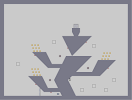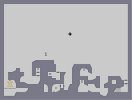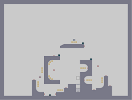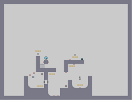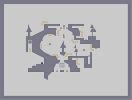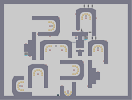Lost As it Stands sigh of relief Request Respect me Call me...NOW!!! (edited)

## Comments

Pages: (0)

### Ha

Sorry I missed this. Make another map.

### Not bad man

not bad. I hope your not leaving numa, if seen this slowly fading away thng before....

duuude!

### looks

like crash bandicoot
HAhahahah

### "a nice furry effect"

wtf. i don't think i have ever z-snapped in my life.

._____.

### Did you intentionally z-snap?

If so it created a nice furry effect. However, the records look like eggs. I think it's a solid 3.

### 4 for just bein

you. Nice job dude, you better get you ass back on here.

hmm..